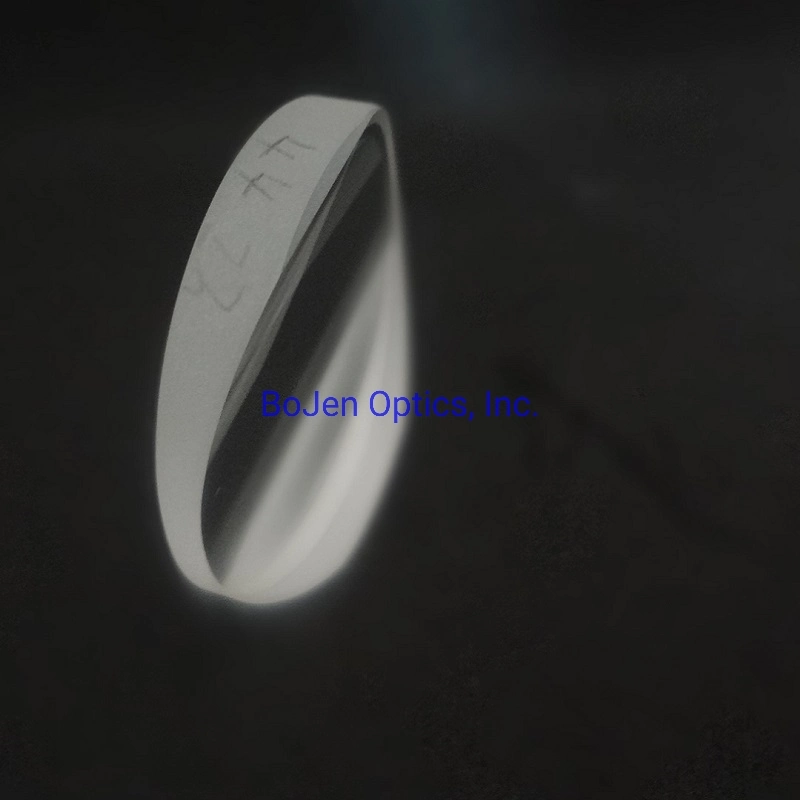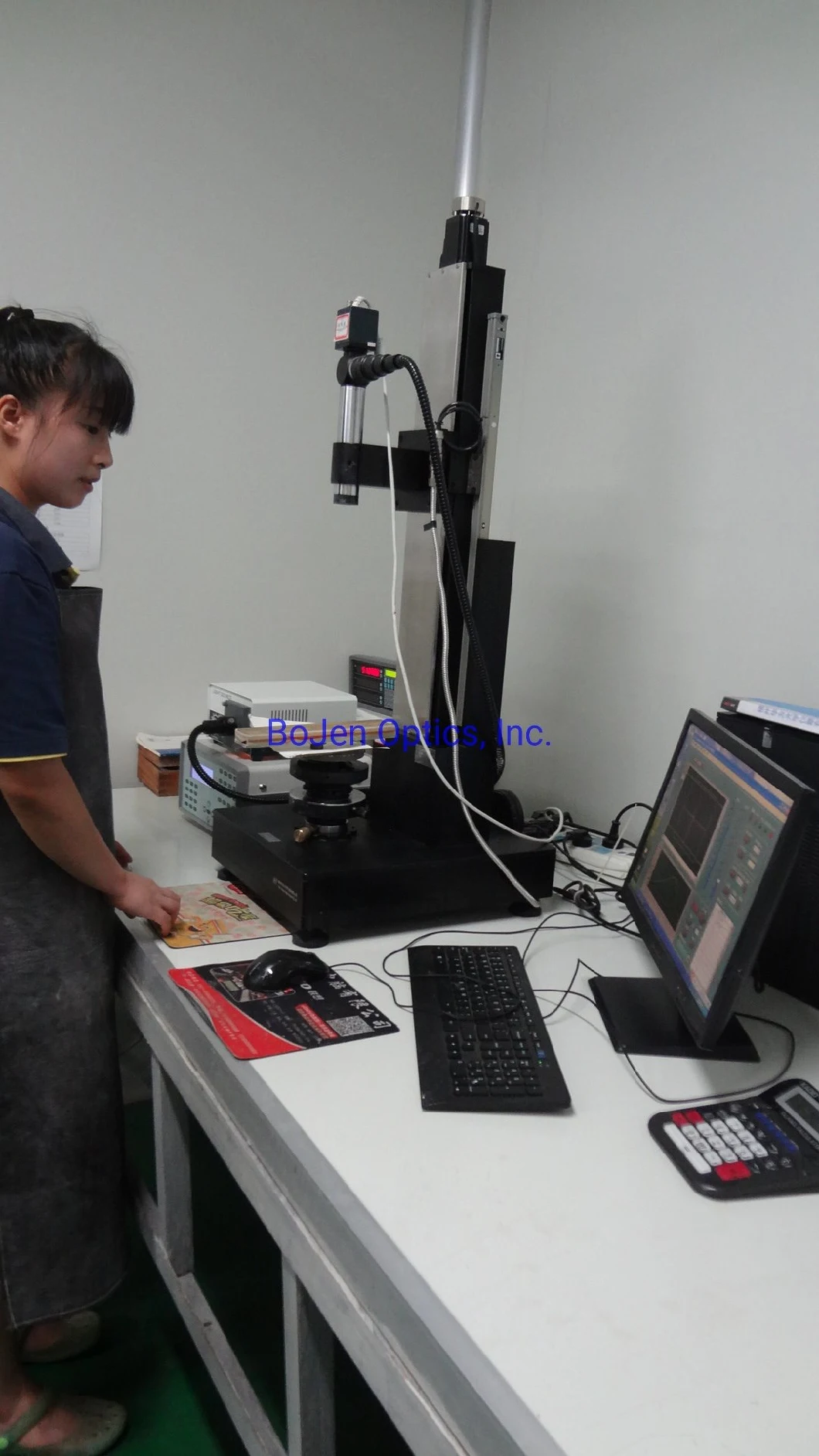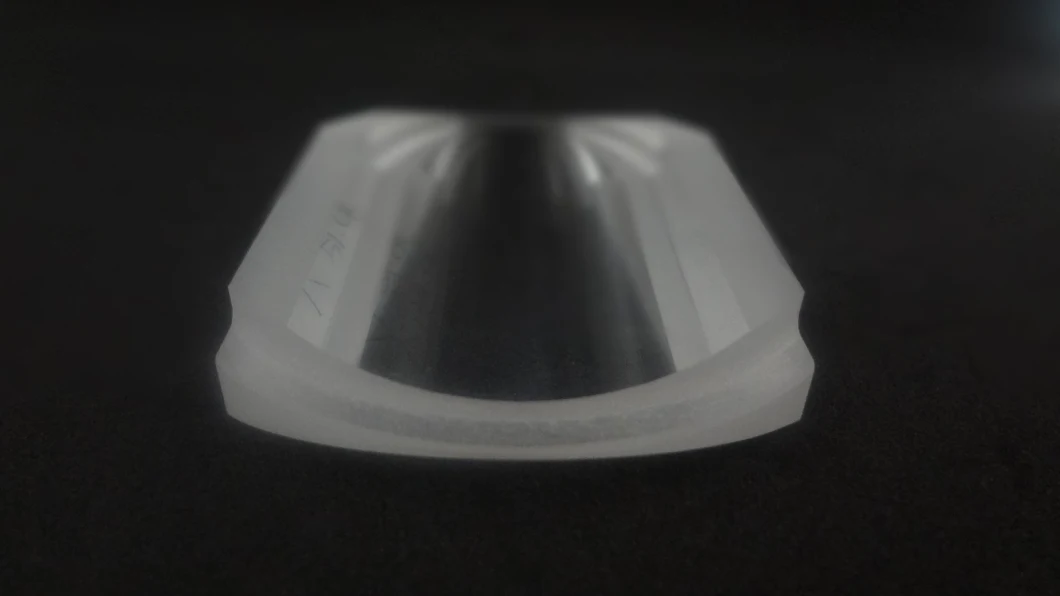# H-K9l UV Fused Silica Plano-Concave Cylindrical Lens

The focal length of each lens can be calculated simply using the following equation:f= R/(n-1),where n is the index of refraction and R1,R2 is the radius of curvature for each surface of the lens.FAQ:1) Can you make design?     We can make a simple design, but it is better to provide us the drawing or detaile

# Featured Products

The focal length of each lens can be calculated simply using the following equation:
f= R/(n-1),
where n is the index of refraction and R1,R2 is the radius of curvature for each surface of the lens.FAQ:

1) Can you make design?
We can make a simple design, but it is better to provide us the drawing or detailed required
specification.
Material,Outer Dimension, Thickness, Dimension tolerace, Surface Quality, Fringe & irregularity,
Centration,  Coating, Quantity?

2) What is the MOQ?
The MOQ is 1pcs, but the price will be very expensive if you just required 1pcs, so we suggest
the MOQ10pcs will be better.

3) What is your production capacity?
We can make the lens from 1pcs better 10pcs to 10,000 per month.

4) What is the material frequently used?
N-BK7 (Chinese type H-K9L)
UV Fused Silica
Optical Glass
Crsytal Silicon etc

5) Do you produce lens by Schott, corning material etc?
We suggest better use Chinese equivalent material.
We can also use Schott or corning material, but the price will be expensive.

6) Can you produce Special shape cyllindrical lens?
Yes, we can make the cylindrical lens by different shape, and hole drilled, edge cutting, edge
stage etc.